#### Chapter 12 Heron's Formula R.D. Sharma Solutions for Class 9th MCQ's

Multiple Choice Questions

Mark the correct alternative in each of the following:

1. The sides of a triangle are 16 cm, 30 cm, 34 cm. Its area is:
(a) 225 cm2
(b) 225√3 cm2
(c) 225√3 cm2
(d) 450 cm2

Solution2. The base of an isosceles right triangle is 30 cm. Its area is
(a) 225 cm2
(b) 225√3 cm2
(c) 225√3 cm2
(d) 450 cm2

Solution

Let ABC be the right triangle in which ∠B = 90°
Now,
base = BC
perpendicular = AB
hypotenuse = AC
BC = 30 cm (given)
△ABC is an isosceles right angled triangle. We know that hypotenuse is the longest side of the right triangle.
So, AB = BC = 30 cm
Area of △ABC = 1/2× base × height
= 1/2× BC × AB
= 1/2× 30 × 30
450 cm2

3. The sides of a triangle are 7 cm, 9 cm and 14 cm. Its area is
(a) 12√5 cm2
(b) 12√3 cm2
(c) 24√5 cm2
(d) 63 cm2

Solution4. The sides of a triangular field are 325 m, 300 m and 125 m. Its area is
(a) 18750 m2
(b) 37500 m2
(c) 97500 m2
(d) 48750 m2

Solution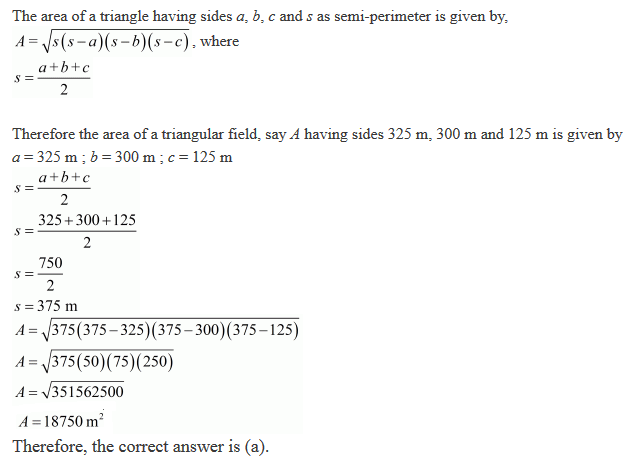5. The sides of a triangle are 50 cm, 78 cm and 112 cm. The smallest altitude is
(a) 20 cm
(b) 30 cm
(c) 40 cm
(d) 50 cm

Solution6. The sides of a triangle are 11 m, 60 m and 61 m. The altitude to the smallest side is
(a) 11 m
(b) 66 m
(c) 50 m
(d) 60 m

Solution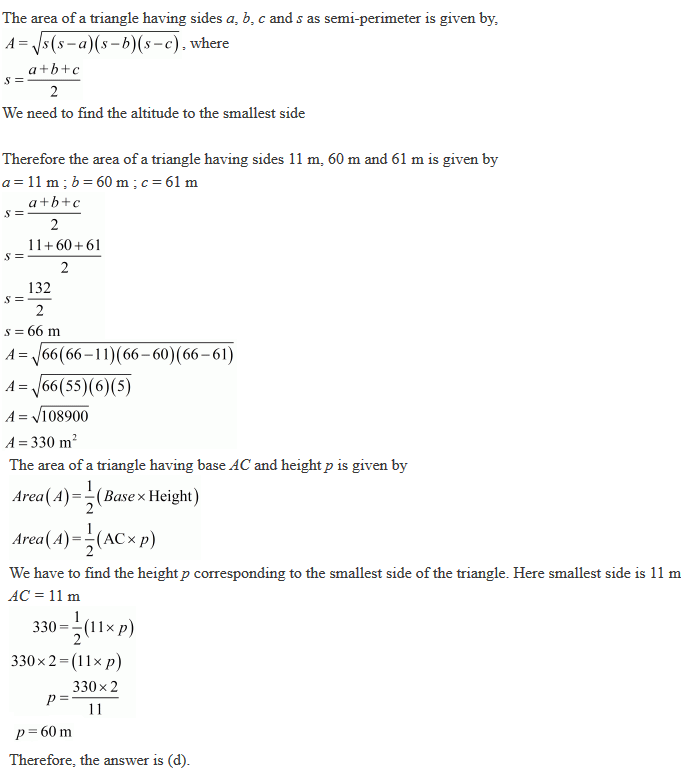7. The sides of a triangle are 11 cm, 15 cm and 16 cm. The altitude to the largest side is
(a) 30√7 cm
(b) 15√7/2 cm
(c) 15√7/4 cm
(d) 30 cm

Solution8. If the area of an isosceles right triangle is 8 cm2, what is the perimeter of the triangle?
(a) 8+√2 cm2
(b) 8 + 4√2 cm2
(c) 4 + 8√2 cm2
(d) 12√2 cm2

Solution

We are given the area of an isosceles right triangle and we have to find its perimeter.
Two sides of isosceles right triangle are equal and we assume the equal sides to be the base and height of the triangle. We are asked to find the perimeter of the triangle
Let us take the base and height of the triangle be x cm.
Area of a isosceles right triangle (A) having base x cm and height x cm is given by:9. The lengths of the sides of ΔABC are consecutive integers. It ΔABC has the same perimeter as an equilateral triangle with a side of length 9 cm, what is the length of the shortest side of ΔABC?
(a) 4
(b) 6
(c) 8
(d) 10

Solution

We are given that triangle ABC has equal perimeter as to the perimeter of an equilateral triangle having side 9 cm. The sides of triangle ABC are consecutive integers. We are asked to find the smallest side of the triangle ABC .
Perimeter of an equilateral triangle, say P having side 9 cm is given by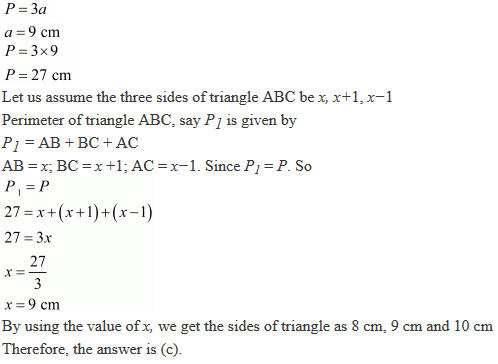10. In figure 12.35, the ratio AD to DC is 3 to 2. If the area of Δ ABC is 40 cm2, what is the area of Δ BDC ?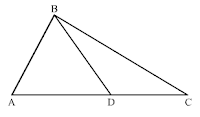Solution11. The base and hypotenuse of right triangular are respectively 5 cm and 13 cm long. Its area is:
(a)25 cm2
(b) 28 cm2
(c) 30 cm2
(d) 40 cm2

Solution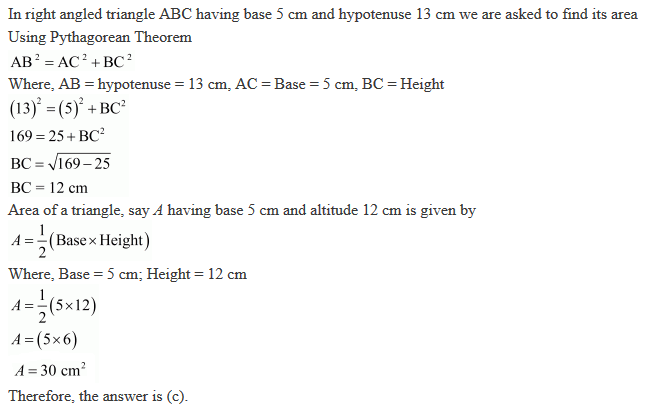12. The length of a median of an equilateral triangle is x cm, then its area is:
(a) x2
(b) √3/2 x2
(c)  x2/√3
(d) x2/2

Solution

We are given the length of median of an equilateral triangle by which we can calculate its side. We are asked to find area of triangle in terms of x
Altitude of an equilateral triangle say L, having equal sides of a cm is given by, where, L = x cm13. The length of each side of an equilateral triangle of area 4√3 cm2 is
(a) 4 cm
(b) 4/√3 cm
(c) √3/4 cm
(d) 3 cm

Solution14. If every side of a triangle is doubled, then increase in the area of the triangle is
(a) 100√2 %
(b) 200 %
(c) 300 %
(d) 400 %

Solution15. A square and an equilateral triangle have equal perimeters. If the diagonal of the square is 12√2 cm, then area of the triangle is
(a) 24√2 cm2
(b) 24√3 cm2
(c) 48√3 cm2
(b) 64√3 cm2

Solution